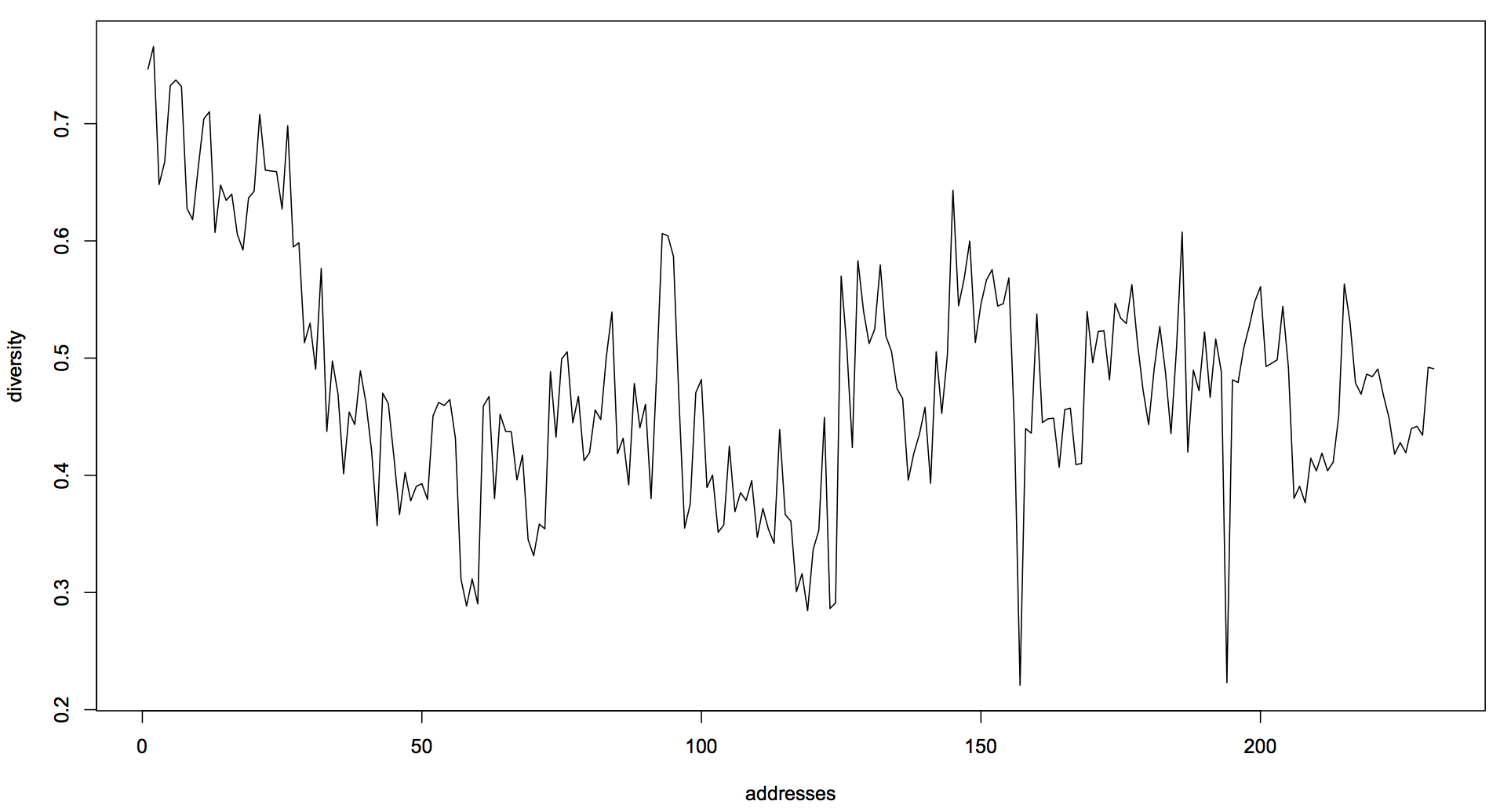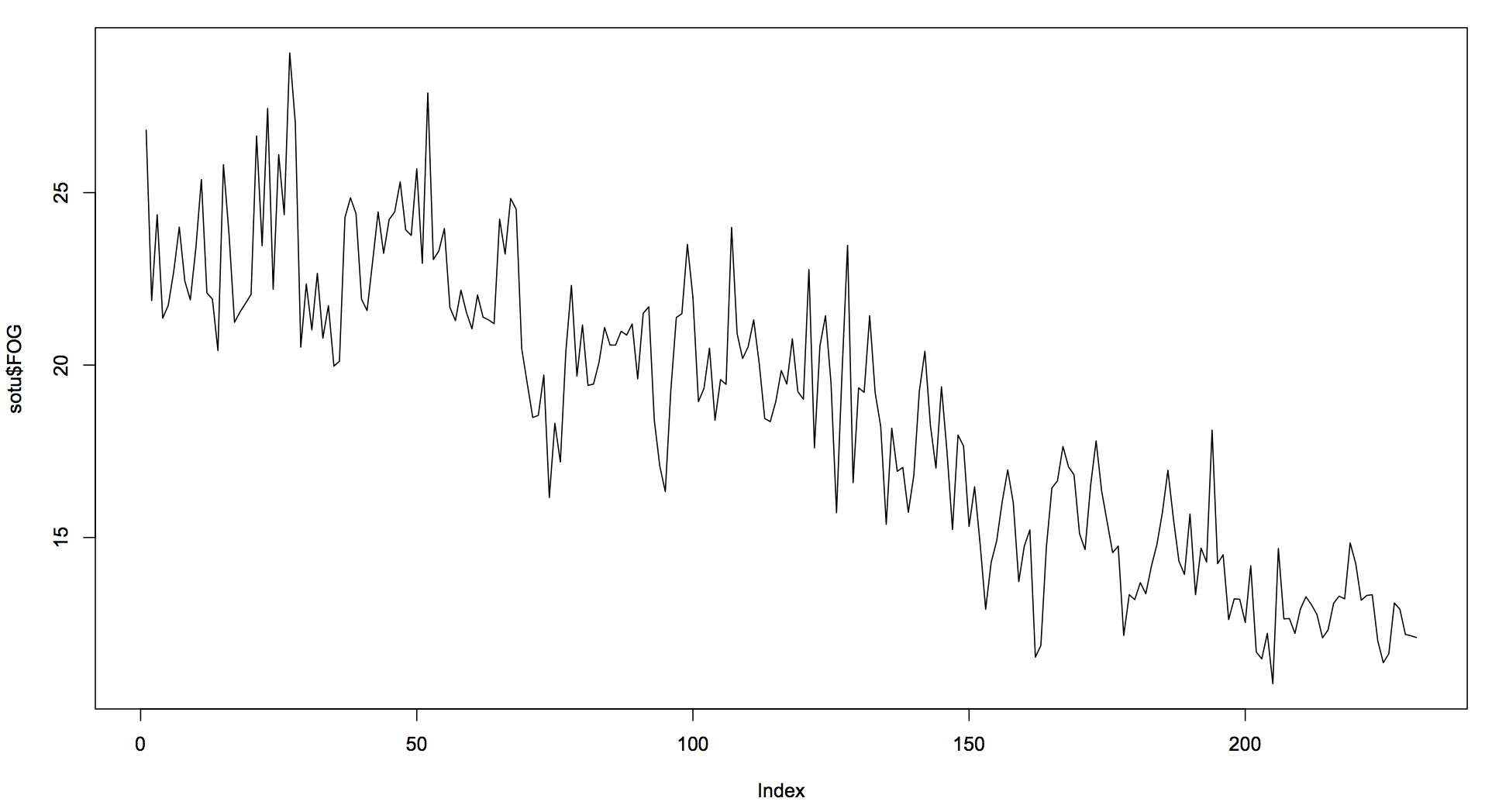# Linguistic Analysis of the State of the Union Addresses

This weekend I harvested 231 State of the Union addresses up to 2017 and put them through NLP processing.

Here are the unigram TF-IDF values, generated with this code of mine (full output: sotu-1-gram).  Each file is named with "YYYYMMDD-Name" format.

``````1. 17900108-Washington.txt
1. licentiousness = 0.0045366833
2. intimating = 0.0045366833
2. 17901208-Washington.txt
1. misemployed = 0.0036363261
2. empowers = 0.0036363261
3. residuary = 0.0036363261
...
230. 20160112-Obama.txt
1. isil = 0.0058471495
2. retrain = 0.0016751325
3. rigged = 0.0013369885
4. brothers = 0.0012484422
5. online = 0.0012484422
6. automated = 0.0008375663
7. cop = 0.0008375663
8. peddling = 0.0008375663
9. clocked = 0.0008375663
10. spilling = 0.0008375663
231. 20170228-Trump.txt
1. obamacare = 0.0043560855
2. oliver = 0.0034848684
3. megan = 0.0034848684
4. susan = 0.0034848684
5. megan's = 0.0034848684
6. jenna = 0.0034848684
7. jamiel = 0.0034848684
8. jessica = 0.0026136513
9. denisha = 0.0026136513
10. shaw = 0.0017424342
``````

And here the bigrams (full output - sotu-2-gram):

``````1. 17900108-Washington.txt
1. work allowed = 0.0161891231
2. inviolable respect = 0.0161891231
3. fund designated = 0.0161891231
4. punish aggressors = 0.0161891231
6. though arduous = 0.0161891231
7. also render = 0.0161891231
9. competent fund = 0.0161891231
10. particularly recommended = 0.0161891231
2. 17901208-Washington.txt
1. case submitted = 0.0132045362
2. national impressions = 0.0132045362
3. northwest side = 0.0132045362
4. shall cause = 0.0132045362
5. attention seems = 0.0132045362
6. uniform process = 0.0132045362
7. peculiarly shocking = 0.0132045362
9. friendly indulgence = 0.0132045362
10. us abundant = 0.0132045362
...
230. 20160112-Obama.txt
1. pass muster = 0.0050289617
2. big question = 0.0050289617
3. unarmed truth = 0.0050289617
4. unconditional love = 0.0050289617
5. respects us = 0.0050289617
6. many issues = 0.0043884723
7. economy contracts = 0.0025144808
8. everybody willing = 0.0025144808
9. new terrorist = 0.0025144808
10. offering every = 0.0025144808
231. 20170228-Trump.txt
1. joining us = 0.0098689435
2. american child = 0.0064590250
3. th year = 0.0049649083
4. rare disease = 0.0049344718
5. incredible young = 0.0049344718
6. megan's life = 0.0049344718
7. jamiel shaw = 0.0049344718
8. recent threats = 0.0049344718
9. jessica davis = 0.0049344718
10. republican president = 0.0049344718
``````

The lexical diversity is shown in the following graph:``````R> df\$file[ which( df\$div == max(df\$div) ) ]
 "17901208-Washington.txt"

R> df\$file[ which( df\$div == min(df\$div) ) ]
 "19460121-Truman.txt"
````````````R> sotu\$Name[ which( sotu\$FOG == max(sotu\$FOG) ) ]